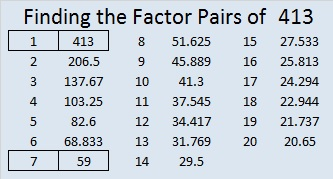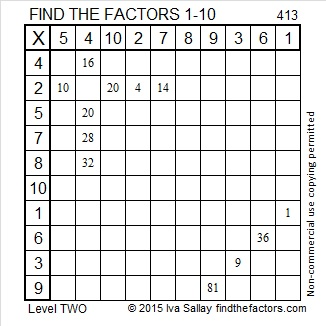# 413 and Level 2

413 can’t be divided evenly by 2, 3, or 5. Can it be divided by 7? Here’s a couple of quick divisibility tricks to try, each requiring the last number to be separated from the rest giving us 41 and 3.

1. Double the 3 and subtract it from 41. That gives us 41 – 6 = 35, a multiple of 7 so 413 can be divided evenly by 7.
2. Multiply the 3 by five and add it to 41. That gives us 41 + 15 = 56, a multiple of 7 as well, so we can draw the same conclusion.

Thank you to abyssbrain for telling me about the second divisibility trick. All of 413’s factors are listed below the puzzle.Print the puzzles or type the factors on this excel file: 10 Factors 2015-03-02

• 413 is a composite number.
• Prime factorization: 413 = 7 x 59
• The exponents in the prime factorization are 1 and 1. Adding one to each and multiplying we get (1 + 1)(1 + 1) = 2 x 2 = 4. Therefore 413 has exactly 4 factors.
• Factors of 413: 1, 7, 59, 413
• Factor pairs: 413 = 1 x 413 or 7 x 59
• 413 has no square factors that allow its square root to be simplified. √413 ≈ 20.3224## 2 thoughts on “413 and Level 2”

1.davescarthin

413 isn’t an a^2 + b^2 as BOTH its prime factors of are of the form 4m+3. Neither can it be of the form a^2 + ab + b^2 so as to be the area (in little triangles) of a triangle on the triangular lattice because its of the form 3n+2. So I don’t think I can make it the basis of a tessellation. Apols if an irrelevant comment.

•ivasallay

I knew it couldn’t be the hypotenuse in a Pythagorean triple, but I didn’t know it couldn’t be the basis of one of your fabulous tessellations. Thank you for the information!

This site uses Akismet to reduce spam. Learn how your comment data is processed.